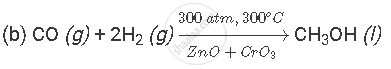Share

# When a Mixture of Gases X and Y is Compressed to 300 Atm Pressure and Then Passed Over a Catalyst Consisting of a Mixture of Zinc Oxide and Chromium Oxide (Heated to a Temperature of 300°C), Then an Organic Compounds Z Having the Molecular Formula Ch4o is Formed. X is a Highly Poisonous Gas Which is Formed in Appreciable Amounts When a Fuel Burns in a Limited Supply of Air; Y is a Gas Which Can Be Made by the Action of a Dilute Acid on an Active Metal; and Z is a Liquid Organic Compound Which Can React with Sodium Metal to Produce Hydrogen Gas. (A) What Are X, Y and Z? (B) Write a Balanced Chemical Equation of the Reaction Which Takes Place When X and Y Combine to Form Z. Indicate the Conditions Under Which the Reaction Occurs. - CBSE Class 10 - Science

#### Question

When a mixture of gases X and Y is compressed to 300 atm pressure and then passed over a catalyst consisting of a mixture of zinc oxide and chromium oxide (heated to a temperature of 300°C), then an organic compounds Z having the molecular formula CH4O is formed. X is a highly poisonous gas which is formed in appreciable amounts when a fuel burns in a limited supply of air; Y is a gas which can be made by the action of a dilute acid on an active metal; and Z is a liquid organic compound which can react with sodium metal to produce hydrogen gas.
(a) What are X, Y and Z?
(b) Write a balanced chemical equation of the reaction which takes place when X and Y combine to form Z. Indicate the conditions under which the reaction occurs.

#### Solution

(a) X is carbon monoxide gas (CO); it is formed when a fuel burns in limited supply of air. Y is hydrogen gas (H2); it is formed by the action of dilute acid on active metal. Z is methanol (CH3OH); it is a liquid organic compound that can react with sodium metal to produce hydrogen gas.Is there an error in this question or solution?

#### APPEARS IN

Lakhmir Singh Solution for Chemistry for Class 10 (2019 Exam) (2018 to Current)
Chapter 1: Chemical Reactions and Equations
Q: 53 | Page no. 23

#### Video TutorialsVIEW ALL 

Solution When a Mixture of Gases X and Y is Compressed to 300 Atm Pressure and Then Passed Over a Catalyst Consisting of a Mixture of Zinc Oxide and Chromium Oxide (Heated to a Temperature of 300°C), Then an Organic Compounds Z Having the Molecular Formula Ch4o is Formed. X is a Highly Poisonous Gas Which is Formed in Appreciable Amounts When a Fuel Burns in a Limited Supply of Air; Y is a Gas Which Can Be Made by the Action of a Dilute Acid on an Active Metal; and Z is a Liquid Organic Compound Which Can React with Sodium Metal to Produce Hydrogen Gas. (A) What Are X, Y and Z? (B) Write a Balanced Chemical Equation of the Reaction Which Takes Place When X and Y Combine to Form Z. Indicate the Conditions Under Which the Reaction Occurs. Concept: Balanced Chemical Equation.
S